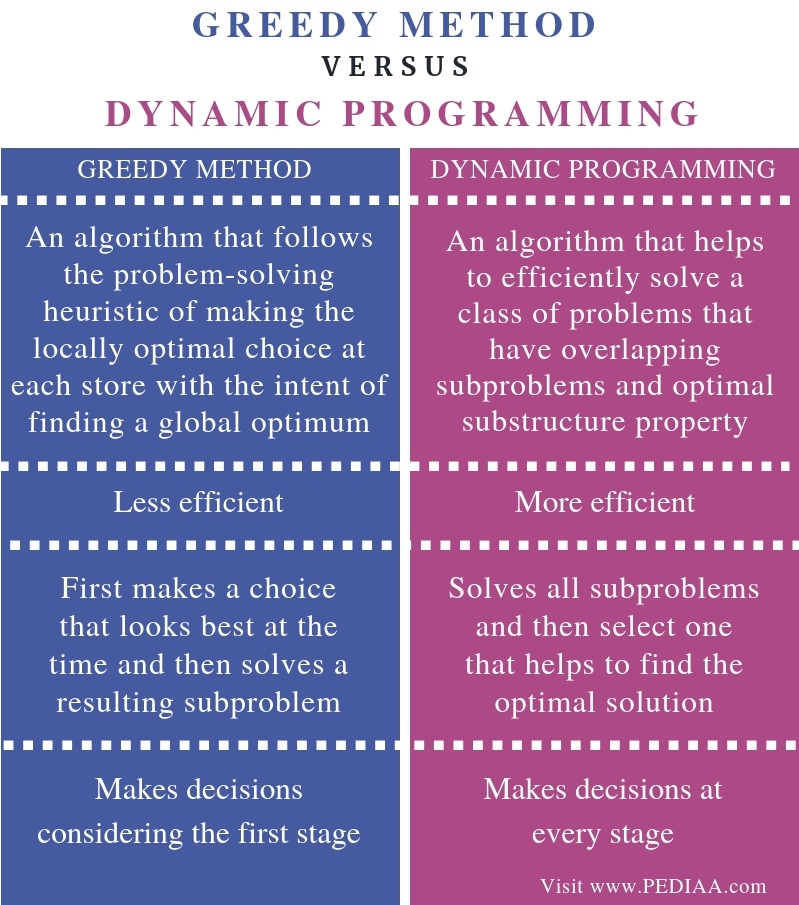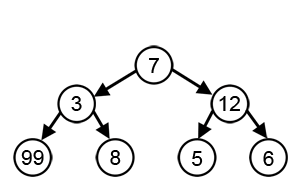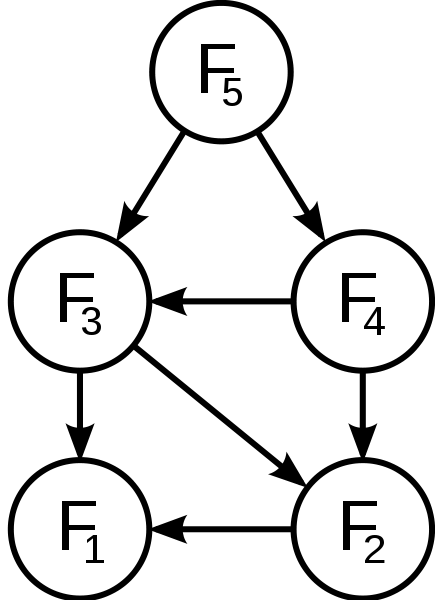# What is the Difference Between Greedy Method and Dynamic Programming

The main difference between Greedy Method and Dynamic Programming is that the decision (choice) made by Greedy method depends on the decisions (choices) made so far and does not rely on future choices or all the solutions to the subproblems. On the other hand, Dynamic programming makes decisions based on all the decisions made in the previous stage to solve the problem.

An algorithm is a systematic sequence of steps to solve a problem. Greedy method and dynamic programming are two algorithms. Both are used to solve optimization problems.

### Key Areas Covered

1. What is Greedy Method
– Definition, Functionality
2. What is Dynamic Programming
– Definition, Functionality
3. What is the Difference Between Greedy Method and Dynamic Programming
– Comparison of Key Differences

### Key Terms

Greedy Method, Dynamic Programming## What is Greedy Method

Greedy method involves finding the best option out of multiple present values. In this method, we consider the first stage and decide the output without considering the future outputs. In other words, the Greedy algorithm solves the problem by considering the best option at that specific moment.Greedy algorithm works if the problem contains two properties as greedy choice property and optimal substructure. It is possible to find a globally optimal solution by creating a locally optimal solution. In other words, creating greedy choices helps to find the optimal solution. Hence, this property is called greedy choice property. Moreover, optimal solutions contain optimal sub solutions. Thus, this property is called optimal substructure.

## What is Dynamic Programming

Dynamic programming involves dividing the main problem into small subproblems. The method stores the results of subproblems and applies them to similar subproblems. Here, storing the answers of subproblems is called memorization. It checks the answers of subproblems and finally comes to a conclusion to find the optimal or best solution. As dynamic programming checks the previous answers and avoids computing the same answer multiple times, it is more efficient.In dynamic programming, the optimal solution to the main problem is within the optimal solution of its subproblems. Furthermore, when there are situations of facing the same subproblems, again and again, it is called overlapping subproblems.

## Difference Between Greedy Method and Dynamic Programming

### Definition

Greedy method is an algorithm that follows the problem-solving heuristic of making the locally optimal choice at each store with the intent of finding a global optimum. Dynamic Programming, on the other hand, is an algorithm that helps to efficiently solve a class of problems that have overlapping subproblems and optimal substructure property. These definitions explain the main difference between Greedy Method and Dynamic Programming.

### Efficiency

Furthermore, a major difference between Greedy Method and Dynamic Programming is their efficiency. The Greedy method is less efficient while the Dynamic programming is more efficient.

### Process

Moreover, an important difference between Greedy Method and Dynamic Programming is that the Greedy method first makes a choice that looks best at the time and then solves a resulting subproblem. Dynamic programming solves all subproblems and then select one that helps to find the optimal solution.

### Decision Making

Method of decision making is yet another difference between Greedy Method and Dynamic Programming. The Greedy method makes decisions considering the first stage while the dynamic programming makes decisions at every stage.

### Conclusion

The decision (choice) made by Greedy method depends on the decisions (choices) made so far and does not rely on future choices or all the solutions to the subproblems. However, Dynamic programming makes decisions based on all the decisions made in the previous stage to solve the problem. That is the main difference between Greedy Method and Dynamic Programming.

##### Reference:

1. “Dynamic Programming Introduction – Javatpoint.” Www.javatpoint.com, Available here.
2. Dynamic Programming | Steps to Design & Applications |, Education 4u, 2 Apr. 2018, Available here.
3. “Greedy Algorithms Introduction – Javatpoint.” Www.javatpoint.com, Available here.
4. “Greedy Algorithm.” Wikipedia, Wikimedia Foundation, 9 Oct. 2018, Available here.

##### Image Courtesy:

1. “Greedy-search-path-example” By Swfung8 – Own work (CC BY-SA 3.0) via Commons Wikimedia
2. “Fibonacci dynamic programming” By en:User:Dcoatzee, traced by User:Stannered – en:Image:Fibonacci dynamic programming.png (Domini públic) via Commons Wikimedia